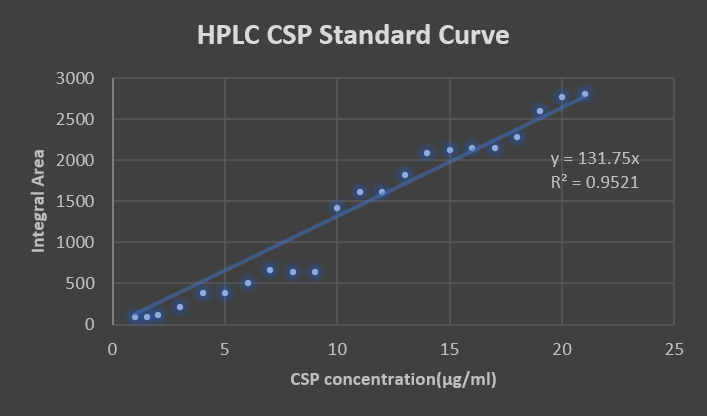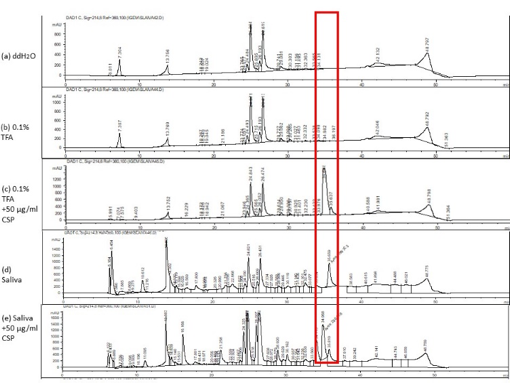# Team:CCU Taiwan/Machine learning

No Sidebar - Helios by HTML5 UP

## Introduction

We use machine learning to generate a model in order to predict user’s risk of caries. We took ICDAS as our standard data and train our model with CSP, lactate, concentration and pH value. In the following section, we will introduce how we preprocess our data for training the model and how we generate the model using scikit learn.

## Data preprocessing

We need three parameters for training the model, which is, concentration of lactate, CSP and pH level. The following will explain how we get those data:

1. Lactate

We use Dimension RxL for a lactate dehydrogenase test:
Then we quantized the concentration of sample by NADH absorbance.

1. CSP

We used HPLC to quantize the concentration of CSP in saliva. We made a standard curve of CSP in HPLC(fig.1), then conducted an experiment to check whether HPLC can exactly analyze CSP in human saliva (fig.2). As for the test, we make sure that the peak of CSP will appear between the time periods of 34 and 35 minute. Next, we injected 150μl filtered saliva into HPLC and gain the actual concentration by calculating on a standard curve.Figure.1 Standard Curve.Figure.2 HPLC can exactly analyze CSP in Saliva. Here is five kind of sample, (a) and (b) is negative control, showing that our solvent and buffer would not cause influence. In (c) we injected excess CSP to find where the CSP peak was. Then we had a filtered saliva test (d) to check the signal situation of HPLC in saliva sample, and here are many peaks. So we injected another saliva sample with excess CSP (e) to make sure which peak in this environment was CSP.

1. pH value

We use commercial pH test paper to evaluate the pH value of saliva.

## Model generation

We use scikit-learn as a tool for generating the machine learning model. We choose the neural network MLP Classifier to train the prediction model. Although the accuracy of the prediction model at first isn’t that accurate due to the lack of data, but we look forward to collecting more data in order to improve the accuracy of the model.